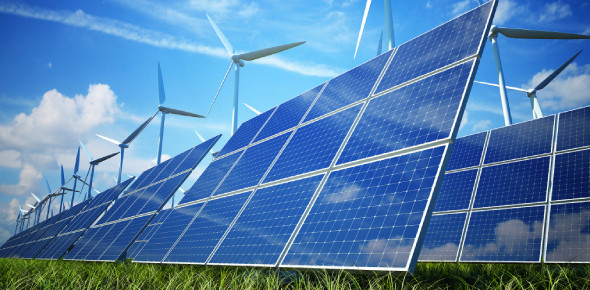# Physics Trivia: Practice Questions On Electricity And Energy!

9 Questions | Total Attempts: 1788Settings.

Related Topics
• 1.
The potential difference required to pass a current 0.2 A in a wire of resistance 20 ohm is ________
• A.

100 V

• B.

4 V

• C.

0.01 V

• D.

40 V

• 2.
Two electric bulbs have resistances in the ratio 1 : 2. If they are joined in series, the energy consumed in these are in the ratio _________
• A.

1:2

• B.

2:1

• C.

4:1

• D.

1:1

• 3.
Kilowatt-hour is the unit of __________
• A.

Potential difference

• B.

Electric power

• C.

Electric energy

• D.

Charge

• 4.
________ surface absorbs more heat than any other surface under identical conditions.
• A.

White

• B.

Rough

• C.

Black

• D.

Yellow

• 5.
The atomic number of natural radioactive element is _________
• A.

Greater than 82

• B.

Less than 82

• C.

Not defined

• D.

Atleast 92

• 6.
From the following statements write down that which does not represent ohm’s law.
• A.

Current / potential difference = constant

• B.

Potential difference / current = constant

• C.

Current = resistance x potential difference

• 7.
Fill in the blanks Potential difference : voltmeter, then:  current __________
• A.

Ammeter

• B.

Voltmeter

• C.

Galvanometer

• D.

Parapmeter

• 8.
Fill in the blanks Power plant : conventional source of energy then solar energy _____. (Non-conventional source)
• A.

Conventional source

• B.

Non-conventional source

• 9.
In the list of sources of energy given below, some of them are wrong. List out the wrong one. Wind energy, solar energy, hydro electric power, tidal energy,
• A.

Wind energy

• B.

Solar energy

• C.

Hydro electric power

• D.

Tidal energy